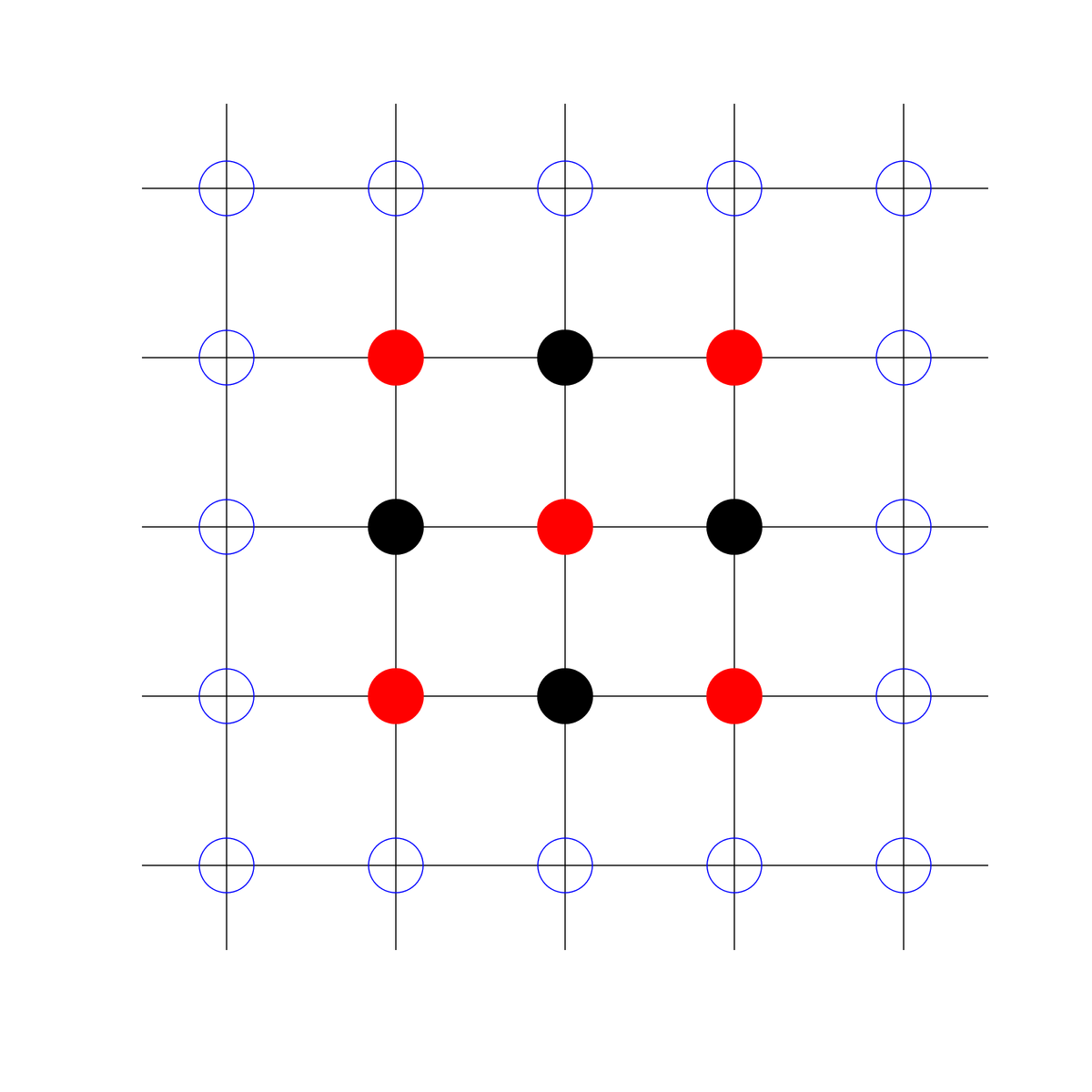## Numerical Methods for Engineers

### University/Institute: The Hong Kong University of Science and Technology## Description

Numerical Methods for Engineers covers the most important numerical methods that an engineer should know. We derive basic algorithms in root finding, matrix algebra, integration and interpolation, ordinary and partial differential equations. We learn how to use MATLAB to solve numerical problems. Access to MATLAB online and the MATLAB grader is given to all students who enroll. We assume students are already familiar with the basics of matrix algebra, differential equations, and vector calculus. Students should have already studied a programming language, and be willing to learn MATLAB. The course contains 74 short lecture videos and MATLAB demonstrations. After each lecture or demonstration, there are problems to solve or programs to write. The course is organized into six weeks, and at the end of each week there is an assessed quiz and a longer programming project. Download the lecture notes: http://www. math. ust. hk/~machas/numerical-methods-for-engineers. pdf Watch the promotional video: https://youtu. be/qFJGMBDfFMY